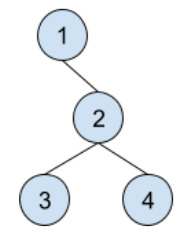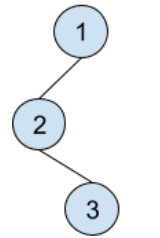New update is available. Click here to update.
Topics

# Icarus and BSTCOUNT

Hard0/120
0 upvote## Problem statement

Icarus is given a binary search tree consisting of ‘N’ nodes. He have to find the different number of permutations of the tree nodes modulo 10^9+7. Where a permutation of a tree is an arrangement of nodes in the original tree such that the structure remains same but there exists at least one position in the permutation whose value is different from original tree.

Output the number of distinct permutations modulo 10^9+7.

Detailed explanation ( Input/output format, Notes, Images )
Constraints :
``````1 <= T <= 10
1 <= N <= 10^5
It is guaranteed that the given input is a binary search tree.

Time Limit: 1 sec
``````
Sample Input 1 :
``````2
4
1 -1 2 3 4 -1 -1 -1 -1
3
1 2 -1 -1 3 -1 -1
``````
Sample Output 1 :
``````1
0
``````
Explanation Of Sample Input 1 :
``````For test case 1 we have,

The input tree:
````````````The different permutations are :

[1, -1, 2, 4, 3, -1, -1, -1, -1]

Hence the answer is 1 % (10^9+7) = 1.

For test case 2 we have,

The input tree :
````````````No other permutations exists for this tree.
Hence the answer is 0 % (10^9+7) = 0.

So, we output 1(true).
``````
Sample Input 2 :
``````2
3
1 2 -1 3 -1 -1 -1
3
1 2 -1 3 -1 -1 -1
``````
Sample Output 2 :
``````0
0
``````Console